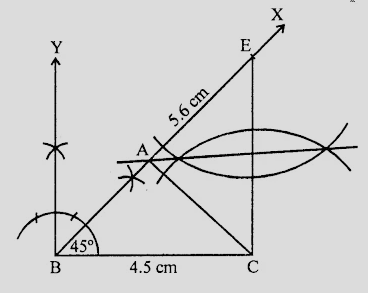# Construct a $Ã¢Ë†â€ ABC$ in which $AB + AC = 5.6\ cm, BC = 4.5\ cm$ and $\angle B = 45^o$.

Given:

A $∆ABC$ in which $AB + AC = 5.6\ cm, BC = 4.5\ cm$ and $\angle B = 45^o$.

To do:

We have to construct the given triangle.

Solution:Steps of construction:

(i) Draw a line segment $BC = 4.5\ cm$.

(ii) At $B$, draw a ray $BX$ making an angle of $45^o$ and cut off $BE = 5.6\ cm$ and join $CE$.

(iii) Draw the perpendicular bisector of $CE$ which intersects $BE$ at $A$.

(iv) Join $AC$.

Therefore,

$\triangle ABC$ is the required triangle.